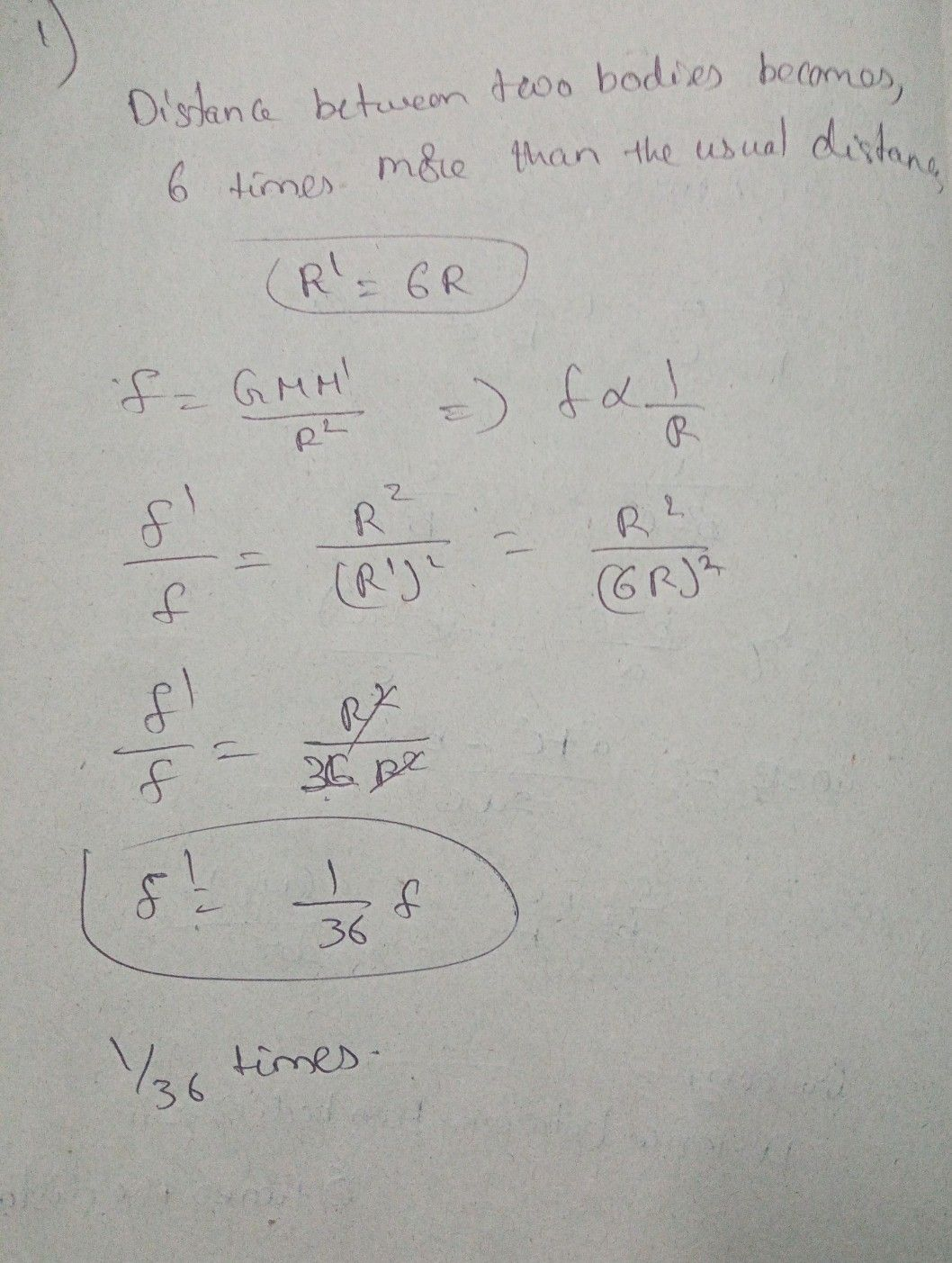Symbol
Problem$\left(0\right)387$ $2$ $0$ $9$ The dis tence betaeen tawo bodies $ba$ $com$ $6$ times mone than the saal distance. $Ths$ the F $becomy$ $\left(0\right)$ 36 times $\left(b\right)$ 6times $c\right)12+imy$ ()'times $a$ $+0^{+i0na}$ force